Next: 2. Distribution Up: 1. Introductory Material Previous: 1. Introductory Material

Subsections

# 1. Introduction

IMPS is an Interactive Mathematical Proof System developed at The MITRE Corporation by W. Farmer, J. Guttman, and J. Thayer. For a technical overview of the system, see .

# 1.1 Overview of the Manual

The manual consists of three parts:

(1)
Introductory Material. This part should definitely be read first. Chapter 3, A Brief Tutorial,'' provides a quick way to start using IMPS.
(2)
User's Guide. This part describes how to use IMPS, particularly, how to build theories.
(3)
Reference Manual.

# 1.2 Goals

IMPS aims at computational support for traditional techniques of mathematics. It is based on three observations about rigorous mathematics:

• Mathematics emphasizes the axiomatic method. Characteristics of mathematical structures are summarized in axioms. Theorems are derived for all structures satisfying the axioms. Frequently, a clever change of perspective shows that a structure is an instance of another theory, thus also bringing its theorems to bear.
• Many branches of mathematics emphasize functions, including partial functions. Moreover, the classes of objects studied may be nested, as are the integers and the reals; or overlapping, as are the bounded functions and the continuous functions.
• Proof proceeds by a blend of computation and formal inference.

## 1.2.1 Support for the Axiomatic Method

IMPS supports the little theories'' version of the axiomatic method, as opposed to the big theory'' version. In the big theory approach, all reasoning is carried out within one theory--usually some highly expressive theory, such as Zermelo-Fraenkel set theory. In the little theories approach, reasoning is distributed over a network of theories. Results are typically proved in compact, abstract theories, and then transported as needed to more concrete theories, or indeed to other equally abstract theories. Theory interpretations provide the mechanism for transporting theorems. The little theories style of the axiomatic method is employed extensively in mathematical practice; in , we discuss its benefits for mechanical theorem provers, and how the approach is used in IMPS.

## 1.2.2 Logic

Standard mathematical reasoning in many areas focuses on functions and their properties, together with operations on functions. For this reason, IMPS is based on a version of simple type theory. 1.1 However, we have adopted a version, called LUTINS, 1.2 containing partial functions, because they are ubiquitous in both mathematics and computer science. Although terms, such as 2/0, may be nondenoting, the logic is bivalent and formulas always have a truth value. In particular, an atomic formula is false if any of its constituents is nondenoting. This convention follows an approach common in traditional rigorous mathematics, and it entails only small changes in the axioms and rules of classical simple type theory .

Moreover, LUTINS allows subtypes to be included within types. Thus, for instance, the natural numbers form a subtype of the reals, and the continuous (real) functions a subtype of the functions from reals to reals. The subtyping mechanism facilitates machine deduction, because the subtype of an expression gives some immediate information about the expression's value, if it is defined at all. Moreover, many theorems have restrictions that can be stated in terms of the subtype of a value, and the theorem prover can be programmed to handle these assertions using special algorithms.

This subtyping mechanism interacts well with the type theory only because functions may be partial. If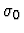is a subtype of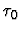, while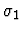is a subtype of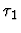, then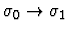is a subtype of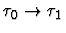. In particular, it contains just those partial functions that are never defined for arguments outside, and which never yield values outside.

## 1.2.3 Computation and Proof

One problem in understanding and controlling the behavior of theorem provers is that a derivation in predicate logic contains too much information.

The mathematician devotes considerable effort to proving lemmas that justify computational procedures. Although these are frequently equations or conditional equations, they are sometimes more complicated quantified expressions, and sometimes they involve other relations, such as ordering relations. Once the lemmas are available, they are used repeatedly to transform expressions of interest. Algorithms justified by the lemmas may also be used; the algorithm for differentiating polynomials, for example. The mathematician has no interest in those parts of a formal derivation that implement'' these processes within predicate logic.

On the other hand, to understand the structure of a proof (or especially, a partial proof attempt), the mathematician wants to see the premises and conclusions of the informative formal inferences.

Thus, the right sort of proof is broader than the logician's notion of a formal derivation in, say, a Gentzen-style formal system. In addition to purely formal inferences, IMPS also allows inferences based on sound computations. They are treated as atomic inferences, even though a pure formalization might require hundreds of Gentzen-style formal inference steps. We believe that this more inclusive conception makes IMPS proofs more informative to its users.

# 1.3 Components of the System

The IMPS system consists of four components.

## 1.3.1 Core

All the basic logical and deductive machinery of IMPS on which the soundness of the system depends is included in the core of IMPS. The core is the specification and inference engine of IMPS. The other components of the system provide the means for harnessing the power of the engine.

The organizing unit of the core is the IMPS theory. Mathematically, a theory consists of a LUTINS language plus a set of axioms. A theory is implemented, however, as a data structure to which a variety of information is associated. Some of this information procedurally encodes logical consequences of the theory that are especially relevant to low-level reasoning within the theory. For example, the great majority of questions about the definedness of expressions are answered automatically by IMPS using tabulated information about the domain and range of the functions in a theory. Theories may be enriched by the definition of new sorts and constants and by the installation of theorems.

Proofs in a theory are constructed interactively using a natural style of inference based on sequent calculus. IMPS builds a data structure which records all the actions and results of a proof attempt. This object, called a deduction graph, allows the user to survey the proof, and to choose the order in which he works on different subgoals. Alternative approaches may be tried on the same subgoal. Deduction graphs also are suitable for analysis by software.

The user is only allowed to modify a deduction through the application of primitive inferences, which are akin to rules of inference. Most primitive inferences formalize basic laws of predicate logic and higher-order functions. Others implement computational steps in proofs. For example, one class of primitive inferences performs expression simplification, which uses the logical structure of the expression , together with algebraic simplification, deciding linear inequalities, and applying rewrite rules.

Another special class of primitive inferences compute with theorems'' by applying extremely simple procedures called macetes. 1.3 An elementary macete, which is built by IMPS whenever a theorem is installed in a theory, applies the theorem in a manner determined by its syntax (e.g., as a conditional rewrite rule). Compound macetes are constructed from elementary and other kinds of atomic macetes (including macetes that beta-reduce and simplify expressions). They apply a collection of theorems in an organized way.

In addition to the machinery for building theories and reasoning within them, the core contains machinery for relating one theory to another via theory interpretations. A theory interpretation can be used to transport'' a theorem from the theory it is proved in to any number of other theories. Theory interpretations are also used in IMPS to show relative consistency of theorems, to formalize symmetry and duality arguments, and to prove universal facts about polymorphic operators. The great majority of the theory interpretations needed by the IMPS user are built by software without user assistance. For example, when a theorem is applied outside of its home theory via a transportable macete, IMPS automatically builds the required theory interpretation if needed.

## 1.3.2 Supporting Machinery

We have built an extension of the core machinery with the following goals in mind:

• To facilitate building and printing of expressions by providing various parsing and printing procedures.
• To make the inference mechanism more flexible and more autonomous. In particular, the set of commands available to users includes an extensible set of inference procedures called strategies. Strategies affect the deduction graph only by using the primitive inference procedures of the core machinery, but are otherwise unrestricted.
• To facilitate construction of theories and interpretations between them.

## 1.3.3 User Interface

The user interface, which is a completely detachable component of IMPS, is mainly designed to provide users with facilities to easily direct and monitor proofs. Specifically, it controls three critical elements of an interactive system:

• The display of the state of the proof. This includes graphical displays of the deduction graph as a tree, TEX typesetting of the proof history, and proof scripts composed of the commands used to build the proof. The graphical display of the deduction graph permits the user to visually determine the set of unproven subgoals and to select a suitable continuation point for the proof. The TEX typesetting facilities allow the user to examine the proof in a mathematically more appealing notation than is possible by raw textual presentation alone. And, with proof scripts, the user can replay the proof, in whole or in part.
• The presentation of options for new proof steps. For any particular subgoal, the interface presents the user with a well-pruned list of commands and macetes to apply. This list is obtained by using syntactic and semantic information which is made available to the interface by the IMPS supporting machinery. For example, in situations where over 400 theorems are available to the user, there are rarely more than ten macetes presented to the user as options.
• The processing of user commands. The commands are then submitted to the inference software, while any additional arguments required to execute the command are requested from the user.

## 1.3.4 Theory Library

IMPS is equipped with a library of basic theories, which can be augmented as desired by the user. The theory of the reals, the central theory in the library specifies the real numbers as a complete ordered field. (The completeness principle is formalized as a second-order axiom, and the rationals and integers are specified as the usual substructures of the reals.) The library also contains various generic'' theories that contain no nonlogical axioms (except possibly the axioms of the theory of the reals). These theories are used for reasoning about objects such as sets, unary functions, and sequences.

# 1.4 Acknowledgments

IMPS was designed and implemented at The MITRE Corporation under the MITRE-Sponsored Research program. Ronald D. Haggarty, Vice President of Research and Technology, deserves special thanks for his strong, unwavering support of the IMPS project.

Several of the key ideas behind IMPS were originally developed by Dr. Leonard Monk on the Heuristics Research Project, also funded by MITRE-Sponsored Research, during 1984-1987. Some of these ideas are described in .

The IMPS core and support machinery are written in the T programming language [18,23], developed at Yale by N. Adams, R. Kelsey, D. Kranz, J. Philbin, and J. Rees. The IMPS user interface is written in the GNU Emacs programming language , developed by R. Stallman.

We are grateful to the Harvard Mathematics Department for providing an ftp site for IMPS.Next: 2. Distribution Up: 1. Introductory Material Previous: 1. Introductory Material